Next: Conserved and Broken Symmetries Up: MANY-NUCLEON SYSTEMS Previous: Effective Interactions (II)

Hartree-Fock method

The Hartree-Fock (HF) approach relies on assuming that the ground state of a many-fermion system can be uniquely characterized by the one-body density matrix (59). There are many ways of deriving the HF equations; the simplest one is to use the variational principle together with the following approximation of the two-body density matrix (60):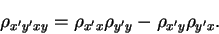(69)

This equation expresses the two-body density matrix by the one-body density matrix, and hence the total energy (58) becomes a functional of the one-body density matrix only,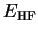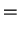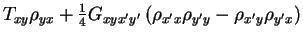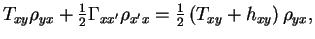(70)

for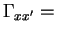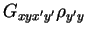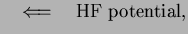(71)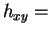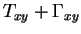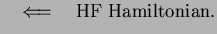(72)

By minimizing the HF energy (70) with respect to the one-body density matrix, one obtains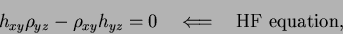(73)

which is usually solved by finding the HF s.p. orbitals that diagonalize the HF Hamiltonian (72),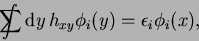(74)

and then constructing the one-body density matrix from these orbitals: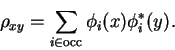(75)

Equations (74) and (75) guarantee that the HF condition (73) is fulfilled (because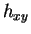and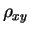are then diagonal in the common basis), so the HF solution is found whenever, for a given set of occupied orbitals,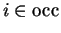, the density matrix self-consistently reproduces the HF potential (71).

From Eq. (75) it is clear that not the real interaction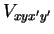, but the effective interaction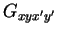, must be used in the HF method. Indeed, when the density-matrix (75) is inserted in the expression for the HF energy (70), one recovers the action of the effective interaction on the two-body product wave functions (61). It is now obvious that the determination of the effective interaction must be coupled to the solution of the HF equations, and performed self-consistently. Namely, for a given effective interaction one solves the HF equations, and the obtained HF orbitals (74) are in turn used in the Bethe-Goldstone equation to find effective interaction. Such a doubly self-consistent procedure is called the Brueckner-Hartree-Fock method.

Modern understanding of the HF approximation is not directly based on the variational method applied to Slater determinants. Certainly, the basic approximation for the two-body density matrix (69) is an exact result for a Slater determinant, but the key element of the approach is expression (70), which states that the ground-state energy can be approximated by a functional of the one-body density matrix.Next: Conserved and Broken Symmetries Up: MANY-NUCLEON SYSTEMS Previous: Effective Interactions (II)
Jacek Dobaczewski 2003-01-27